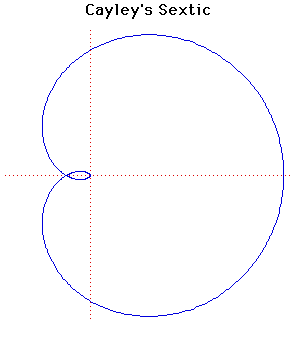# Curves

### Cayley's SexticCartesian equation:
$4(x^{2} + y^{2} - ax)^{3} = 27a^{2}(x^{2} + y^{2})^{2}$
Polar equation:
$r = 4a \cos^{3}( \theta /3)$

### Description

This was first discovered by Maclaurin but studied in detail by Cayley.

The name Cayley's sextic is due to R C Archibald who attempted to classify curves in a paper published in Strasbourg in 1900.

The evolute of Cayley's Sextic is a nephroid curve.

### Associated Curves

Definitions of the Associated curves